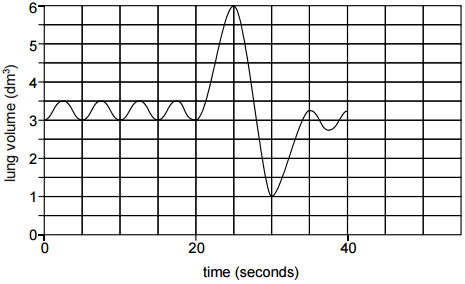# Respiration graph

The graph shows the volume of a subject’s lungs measured over a period of 40 seconds.Determine the total volume of air breathed in during the 40 seconds (give your answer to the nearest 0.25dm3).

A. 7.75 dm3
B. 8 dm3
C. 8.5 dm3
D. 8.75 dm3
E. 9.10 dm3

How to interpret this type of graphs?

this is how i would solve this question

each part contains 0.5 dm3
by calculating 4*0.5 +6 0.5+40.5 +almost 0.5 =7.75
let me know if there is any error

2 Likes